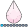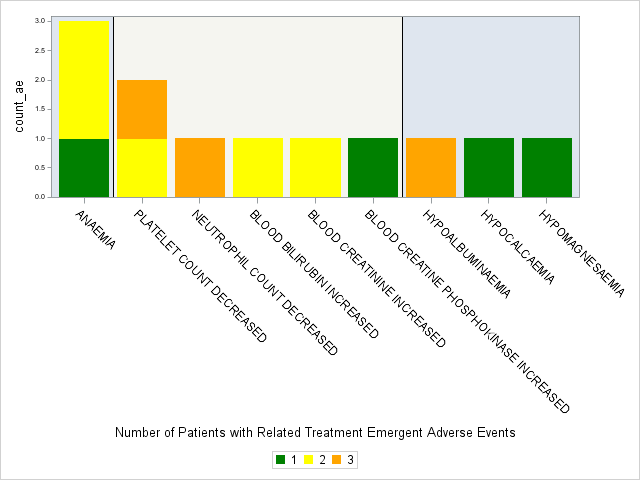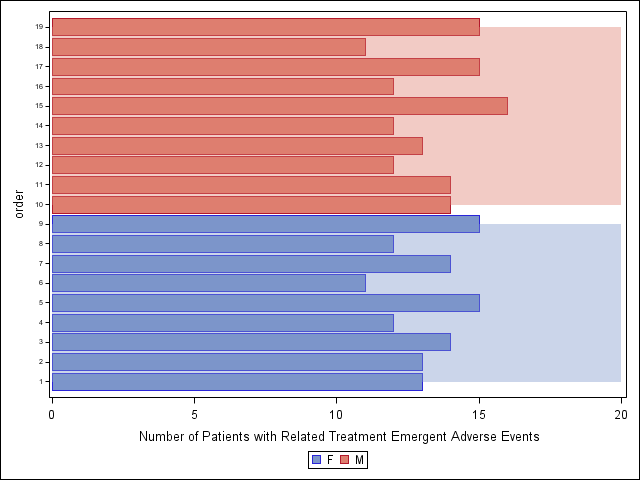djrisks
Barite | Level 11

## Blockplot on y-axis

Hello,

I have a graph that shows the number of adverse events and the severity of the event for a few terms. The terms are split by System Organ Class which is denoted by the bands. I would like to be able to use hbarparm instead of vbarparm, and therefore would like to use the blockplot on the y-axis, however so far I can only make the blockplot work with the x-axis. My main aim is to be able for someone to tell which system organ class the adverse events are related too, and so I thought an easy way to do this was to shade the background.

Attached below is my image. Essentially, I'd like to rotate the image 90 degrees. Have you got any ideas please?Below is the SAS code I have used so far, altough, there is no data.

``````proc sgplot data = count_max4 dattrmap=myattrmap;
block x = AECTC block = aesoc / filltype=alternate novalues;
vbarparm response = count_ae category = AECTC / group = max_aetoxgrn groupdisplay = stack grouporder=ascending attrid = myid name = "leg";
keylegend "leg" / down = 1;
xaxis /*values = (0 to 8 by 1)*/ type = discrete label = "Number of Patients with Related Treatment Emergent Adverse Events";
yaxis valueattrs = (size = 5);
format group grp.;
run;``````

Thank you,

Kriss

1 ACCEPTED SOLUTION

Accepted Solutions

## Re: Blockplot on y-axis

Hi Kriss,

how it's going? I think that the bandplot could not be used with a axis different to the X.

I know that is not the best way, but could you pas the X-axis to numeric, and after that rename the axis with discrete ticklist and use something like

``````
proc template;
define statgraph sgplot_blockplot;
begingraph /;
layout overlay / xaxisopts=( Label="Number of Patients with Related Treatment Emergent Adverse Events" ) yaxisopts=( TickValueAttrs=( Size=5)  );

BarChartParm X=order Y=Age /  orient=horizontal Group=Sex LegendLabel="Age" NAME="leg" groupdisplay=cluster grouporder=ascending;
bandplot y=order limitupper=top
limitlower=down /group=SEX DATATRANSPARENCY=0.6 DISCRETEOFFSET=0.5; ;
DiscreteLegend "leg" / Location=Outside down=1 order=columnMajor;
endlayout;
endgraph;
end;
run;

proc sort data=sashelp.class out=class;
by sex name;
run;

data Class;
set Class;
order=_N_;
top=20;
down=0;
run;

proc sgrender data=class template=sgplot_blockplot;
run;``````4 REPLIES 4

## Re: Blockplot on y-axis

Hi Kriss,

how it's going? I think that the bandplot could not be used with a axis different to the X.

I know that is not the best way, but could you pas the X-axis to numeric, and after that rename the axis with discrete ticklist and use something like

``````
proc template;
define statgraph sgplot_blockplot;
begingraph /;
layout overlay / xaxisopts=( Label="Number of Patients with Related Treatment Emergent Adverse Events" ) yaxisopts=( TickValueAttrs=( Size=5)  );

BarChartParm X=order Y=Age /  orient=horizontal Group=Sex LegendLabel="Age" NAME="leg" groupdisplay=cluster grouporder=ascending;
bandplot y=order limitupper=top
limitlower=down /group=SEX DATATRANSPARENCY=0.6 DISCRETEOFFSET=0.5; ;
DiscreteLegend "leg" / Location=Outside down=1 order=columnMajor;
endlayout;
endgraph;
end;
run;

proc sort data=sashelp.class out=class;
by sex name;
run;

data Class;
set Class;
order=_N_;
top=20;
down=0;
run;

proc sgrender data=class template=sgplot_blockplot;
run;``````djrisks
Barite | Level 11

## Re: Blockplot on y-axis

Hi Antonio,

Yes it's good thanks, I hope you're well.

Thank you for this solution, I was thinking of the band plot too, but knew it worked with numeric values. This is a great workaround! Nice 🙂 Thank you.

KrissJay54
Meteorite | Level 14

## Re: Blockplot on y-axis

Antonio has proposed a good solution. If you wan to cover the edge bar widths, then another way is to use reference lines for each set of bars.  In this case only one set is needed.  But this could be extended for multiple sets using alternate colors or different colors like a BLOCKPLOT.  Names can be shown using YAXISTABLE.

proc sort data=sashelp.class out=class;
by sex name;
run;

data Class;
set Class;
id=_n_;
if sex="M" then refid=id;
run;

proc sgplot data=class;
refline refid / lineattrs=(thickness=16) transparency=0.5;
hbarparm category=id response=age / group=sex;
yaxistable name / position=left location=outside valuejustify=right;
yaxis display=(novalues noticks nolabel);
xaxis offsetmin=0;
run;djrisks
Barite | Level 11

## Re: Blockplot on y-axis

Thank you for this Sanjay, this really helps! 🙂

Discussion stats
• 4 replies
• 1243 views
• 4 likes
• 3 in conversation